# A glimpse of the maths behind Optimization AlgorithmsBy Reina Kousaka, Unsplash licence.

Ok, let’s start…

(∩｀-´)⊃━☆ﾟ.*･｡ﾟ

End…

No? Ok… ¯\_(ツ)_/¯ We need to dive a bit more into maths…

The goal of this article is to provide a basic understanding of the maths behind common types of optimization algorithms. I used both my notes from the DSTI and other sources like papers and blog posts. The links are provided.

We will start from vanilla fixed step gradient descent, for purely education purpose, and pile-up concepts until we get to the widely used Adam optimizer. By the end, we open on some other promising algorithms.

Notations:

• J: ℝⁿ → ℝ is the cost function, we are looking for its minimum.
• x = (x₁, …, xₙ) is a vector of the space ℝⁿ
• x* is our notation for the minimum.
• xₖ is the value of x at the kᵗʰ iteration of the gradient descent.

If you want a refresher on mathematical formulas such as Euler equality, you may find it on this article: “A cheatsheet of mathematical formulas for fundations of Continuous Optimization”.

# 1/ Minimization without constraints

We are looking for inf J(x) on ℝⁿ. J is supposed to be alpha convex and gateaux derivable. Then, by Euler equations, J’(x*) = 0 has a unique solution, that is the infimum.

We want an algorithm that converge to x*. k is the number of iterations. xₖ is the value of x at the kᵗʰ iteration. It is supposed to converge to x*, at least, when k tends to infinity. This mean that we want |xₖ₊₁ — xₖ| to be as large as possible. We note the increment vector yₖ = xₖ₊₁ — xₖ. So that, each yₖ show the direction and the length of the move between two increments.

Using Taylor series we can write:

With ε(yₖ) that converge to 0 when yₖ does.

Since J’(xₖ;yₖ) =∇J(xₖ)⋅yₖ we want to maximise |∇J(xₖ)⋅yₖ|.

By Cauchy Schwarz inequality |∇J(xₖ)⋅yₖ| ≤ ∥ ∇J(xₖ) ∥.∥ yₖ ∥. This higher value is reached when ∇J(xₖ) and yₖ are collinear. We can also notice that the increment vector yₖ is moving to the opposite direction from the gradient (we are descending and the gradient is climbing), so we maximize the dot product when yₖ = -ρₖ ∇J(xₖ), with ρₖ ≥ 0. Since xₖ₊₁ = xₖ + yₖ :

We are basically saying that we will move towards the opposite direction of the gradient by a factor ρₖ of the gradient norm. That’s pretty well what we call a gradient descent.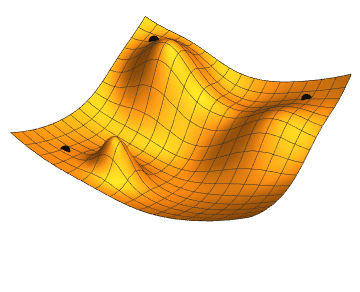An illustration of the gradient descent on ℝ² — By Jacopo Bertolotti — Wikimedia Commons — C0

All the following story is about “how to choose ρₖ so that we converge and, if possible, so that we converge as fast as possible.

## 1.1/ Fixed step algorithm

This is the most “mathematically simple” algorithm, while not a very performant one. It may be interesting to understand the mathematical reasoning behind this simple example. So, let’s dive a bit into it…

Here, we simply choose a fixed value of ρₖ, that we will therefore call ρ.

We are working under the previous hypothesis. J is alpha-convex and Gateaux derivable.

We will also require that J(xₖ) is Lipschitz continuous. By definition of Lipschitz continuity:

M is the maximal slope of the function. Lipschitz continuity mean that J(xₖ) has a slope in every point and that this slope does not tend to infinity. So, it does not tends to become vertical.

Let’s go see what happen at the infimum, where J’(x*) = 0. We take the vector of the last increments : zₖ = x*xₖ.

By replacing into the descent equation, we get:

Let’s compute its norm:

Then insert both terms from:

• Alpha-convexity: (xₖ-x*)(∇J(xₖ)-∇J(x*)) ≤ α ∥ xₖ — x*
• Lipschitz continuity : ∥ ∇J(xₖ) — ∇J(x*) ∥ ≤ M ∥ xₖ — x*

Using the max of quadratic function, we have then:

The condition for convergence is:

In practice, we don’t want to compute alpha and M, so that, will just take a small enough value for rho and test with it.

## 1.2/ Gradient algorithm with optimal step

In this algorithm, we will also maximize the progress value of the step: J(xₖ₊₁)-J(xₖ), to find out the optimal value of rho.

The optimal step gradient algorithm converges for any J that is:

• Gateaux-derivable
• Alpha-convex
• Lipschitz continuous
• … on every bounded subset.

Except on “nice” cases, it usually takes an infinite number of loops to converge. We stop the calculus when it is good enough.

In some cases, we may consider that we have a near quadratic function, where things are easier to calculate. With A definite, positive, symmetric:

We progress by taking normals to the previous direction and stopping when we tangent an isoline ellipse, what mean we would go too far if we continued.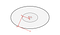Own work released under CC BY 3.0 — Download source

## 1.3/ Conjugate gradient algorithm

The algorithm converges in n step, where n is the dimension of the space. In practice rounding errors prevents the exact convergence in simulations, yet it remain faster than previous methods.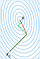Comparing conjugate gradient (in red) with optimal step gradient algorithms. Drawing by Oleg Alexandrov released under Public Domain on Wikimedia Commons.

On quadratic functions:

The difference with optimal step algorithm is that we change one dimension of space to transform the isoline ellipse into a circle and then can target directly at the center. Then we need to repeat for each dimension of the space.

There, not only the successive gradients are orthogonal, but also, all are linearly independent.

If we take dᵢ the direction of the gradient at the iᵗʰ iteration, the algorithm is the following:

One of the common uses of this algorithm is to solve linear systems in the form Ax = b, by transforming it into a quadratic function.

## 1.3/ Newton method

Newton method is a well know approach to find the root of a function. Let’s see how it works in the scenario (that is not minimization yet).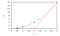By Paul Breen — Wikimedia Commons — C0

With f: ℝⁿ → ℝⁿ, we get the root using its Jacobian matrix ∇f and by solving the linear system on the second line:

For minimization we are looking for a root of the gradient of J. So we take it as: f = ∇J. Then, the Jacobian matrix ∇f becomes the Hessian matrix of J. Such that ∇f = ∇²J. We need to solve:

In practice, since computing the Hessian is costly, we do it only at some steps, keeping it constant otherwise. When we are close to the solution, keeping the Hessian constant is a good approximation.

Often, on high order optimizations, like on neural networks, computing the Hessian is so costly that the method becomes ill suited.

# 2/ Minimization with constraints

## 2.1/ Fixed step gradient algorithm with projection

With restrict the set on which we are looking for the minimum and call it K.

This is the same as the fixed step gradient algorithm, except that, when xₖ is not on K, we will make a projection of the result of the iteration equation, so that xₖ₊₁ come back to K.

It may not always be easy to find out what projection method to use.

## 2.2/ Penalty method.

J: ℝⁿ → ℝ is the function to minimize and F: ℝⁿ → ℝᵐ is the vector function of the constraints such that F(x)=0. We introduce a cost function:

Then we can solve on ℝⁿ, since any point out of {x s.t. F(x)=0} will be strongly penalized.

In practice, if epsilon is too small the problem become ill conditioned.

## 2.3/ Identification of the Lagrangian saddle point with Usawa’s algorithm.

this is once again the fixed step gradient algorithm, but we inject a Lagrangian in the method. J is the function to minimize. F(x) is the vector of the conditions. J and F are considered as convex.

Uzawa algorithm can be quite slow. We can also add a penalty term called augmented Lagrangian that is square, alpha-convex and converge better than linear terms.

# 3) Practical implementations

## 3.1) Stochastic gradient descent

When you compute the gradient on a dataset of observations, take the mean of the gradient of each observation. This can be pretty costly.

With stochastic gradient descent, at each step, you take a random subset of the data to compute the gradient. The resulting gradient may be quite bad on noisy data, but this is way less costly and enable us to do it many more times, on smaller increments. This makes gradient descent scalable on big data sets.

Stochastic gradient descent update vectors tends to be quite noisy individually, and some can even take the opposite direction of the slope. Resulting in noisy, yet converging performance curve.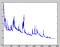Joe pharos — Wikimedia — Released under GFDL

The generated noise can be problematic near to the minimum. Slowly decreasing the learning rate help this algorithm to perform well.

## 3.2) Mini batch gradient descent

In practice, a nice variation is mini batch gradient descent. We cut the data into a finite number of batches and rotate the computation of the gradient on each batch. This reduce the variance of the parameters update end enable more efficient calculus techniques to be used.

## 3.3) Adding momentum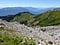Guilhem Vellut — on Flickr — CC BY 2.0

Think of a ball rolling through the chaotic slope of a mountain. A lightweight foam ball is more likely to get perturbed by the rocks, while a heavy steel one is more likely to get straight to the main direction of the slope. This is due to a higher inertia.

Momentum act somewhat similarly, while the equations can’t be compared. There, the issue is not rocks, it is the random changes of direction of the update vector that is due to the stochastic process noise.

Momentum adds a fraction of the previous update vector to the current one. This can be written:

γ can be quite high, as 0.9 is a common value.

Noise is specifically an issue in the direction of low curvatures. Momentum helps to learn the direction of low curvature, without being unstable on the directions of high curvature.

This is very helpful in ravines, since it avoid that the nervous ball jump from wall to wall and help it to stay focused on the lower slop at the bottom.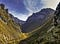By Atlantios — on Pixabay — C0

## 3.4) Nesterov accelerated gradient

Let’s have a look at the previous momentum equation:

What we are doing is computing the gradient on the current point and adding a strong component in the direction of the previous update vector.

Let’s suppose that the new gradient is small compared to the previous update vector, it has little influence on the process. There, this system is a bit brutal… This is an issue when the slope slow down as we approach the minimum, for example.

Reducing γ is a solution for this specific issue, but this will lower the performance on the original issue that is managing the noise created by the stochastic process. Overall, this is not a good solution.

Ilya Sutskever, from OpenIA, had a better idea. Getting inspiration from Nesterov method of optimizing convex function, he suggested to add the fraction of the previous update vector directly inside the gradient. So that, first, we move in the direction of the previous update vector, then we can correct using the gradient of the actual point where we landed, instead of using the gradient of the original point where we started that is no longer up to date.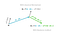Own work released under CC BY 3.0 — Download source

The step’s equation we had seen before become:

You can see a complete implementation of Nesterov method on “Gradient Descent With Nesterov Momentum From Scratch”, by Jason Brownlee.

## 3.5) Adaptive Subgradient Methods (Adagrad)

Until now, the learning rate was the same for each of the parameters. It may sound a good idea to adapt it according to each parameter.

Mathematically a parameter is a coordinate xᵢ of x ∈ ℝⁿ, with i ∈ {1, …, n}. Practically, it is a column of the dataframe.

Let’s say that some of our columns are sparse. As an example, if each column is a word in a Natural Language Processing algorithm, some words, like “Adaptative”, may occur rarely. These are called rare features.

However, in our current article, the word “Adaptative” carry way more sense that the word “Gradient”, that is very frequent. For example, “Adaptative” maybe a good feature if we want our NLP program to guess what algorithm we are speaking about.

The idea of Adagrad is to:

• associate a lower learning rate to frequent features,
• associate a higher learning rate to more rare features.

Adagrad it therefore especially relevant with sparse datasets where some features occur rarely and carry lots of sense.

For the time being, this mean that we have exactly the same step equation as before, yet we think “column wise”, each column having a specific value ρᵢ of the learning rate.

Adagrad defines a diagonal matrix Gₖ, that takes the sum of the subsequent gradients in its diagonal elements. It is used to apply a decay on the learning rate over time:

More details on Adagrad on the original paper or “An introduction to AdaGrad” by Roan Gylberth.

## 3.6) RMSProp and Adadelta, resolving Adagrad’s radically diminishing rates

The weakness of Adagrad is that the accumulation term Gₖ continue to grow over time, eventually resulting in a very small learning rate, only limited by epsilon, that cannot be too big at the start.

RMSProp and Adadelta were created to tackle this issue.

Adadelta and RMS Prop restrict the fixed the number of past gradients taken in account to a fixed windows w < k. Then, Gₖ is more a local estimate using the recent gradients.

There is a “subtlety”: since it may be inefficient to store w previous squared gradients, the implementation is done as an exponentially decaying average of the squared gradients.

RMS Prop equations look like this:

Once again, beta can be quite high as a classical value is 0.9.

Adadelta goes further, completely replacing the rho term by a an exponentially moving average of the squared update vectors:

We can notice that there is some similarities with the momentum previously seen. This, indeed, the idea.

With Adadelta, there is no need to provide a learning rate parameter.

More details are available in:

## 3.7) Adam: Adaptive Moment Estimation

Adam is often used as the “default” optimizer for gradient descent in neural networks, since it performs well in a wide variety of situations.

Adam is similar to AdaGrad and RMSProp.

According to the original paper:

Some of Adam’s advantages are that the magnitudes of parameter updates are invariant to rescaling of the gradient, its stepsizes are approximately bounded by the stepsize hyperparameter, it does not require a stationary objective, it works with sparse gradients, and it naturally performs a form of step size annealing.

Adams take the following parameters, with the following default values proposed by the authors:

• α = 0.001
• β₁ = 0.9
• β₂ = 0.999
• ε = 10⁻⁸

Adams uses the first moment (expected value) mₖ and the second moment (variance) vₖ estimates of the gradient. We note that the square of the gradient represents the element-wise square, not its norm squared.

However, since m and v and initiated at 0 and since the β₁ and β₂ constants are near to 1, these terms are biased towards zero. They grow difficulty during the initial steps. Then, Adam compute a bias-corrected moment estimate by dividing by 1-βᵏ, that is small initially and grow nearer to 1 over iterations. This artificially boost the moments during the first iterations.

Then, we can write Adam’s update function as:

The authors remarks that the ratio m/√v is somewhat similar to a signal-to-noise ratio (SNR). The higher the uncertainty about the direction of the minimum, the smaller the SNR ratio, then, the smaller the step size. Considering that the SNR becomes closer to zero near a minimum, this makes it a desirable property since this permits a smoother approach of the minimum, limiting oscillations.

More details on Adam’s original paper.

## Other algorithms…

We will stop here, but there is tons of other algorithms. Here are some interesting ones:

• In the same paper, Adam authors propose Adamax. Instead of using the second order estimate, it uses the ℓ∞ norm, that is more stable than ℓ1 and ℓ2 norms.
• NAdam, that combines Adam and Nesterov accelerated gradient.
• With Adams, if we have batches that are way more informative than the other, they don’t get enough boost. AMS Grad thus take the maximum of the two past squared gradients: vₖ₊₁ = max(vₖ₊₁,vₖ)
• In neural networks, with Adam, ℓ2 regularization plays a role in penalizing high weights. The idea is that high weights are likely to be overfit, unless this is consistent over time. AdamW decouple weight decay from the gradient update.
• QHAdam replace both momentums in Adam with quasi hyperbolic terms. This makes possible to parametrize the function to look like Adam, NAdam, or others.
• YellowFin provides an automatic tuner for momentum and learning rate. According to the paper, there is a significant gain over Adam.
• Demon (Decaying Momentum) The authors observe that a high momentum (like 0.9) is nice to start with, while by the end it may penalize the model. Like if the ball was moving on a minimums’ plateau without finding the most optimal spot. Moreover, diminishing this parameter when it is less needed, makes the model less dependant on parameters tuning. Demon can be implemented in vanilla SGD with momentum, as well as in Adam.

# Read further:

Publications in French & English about design, innovation and programming.